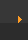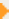## Stoichiometry: Introduction to Moles (1 of 4)

 Next pageThe mole is a frequently used unit for the amount of atoms, molecules, ions, etc.

One mole of any element consists of 6.02 x 1023 particles (e.g. atoms, molecules, etc.).

6.02 x 1023 is known as Avogadro's Number.

The mass of one mole of an element is called the Gram Atomic Mass (GAM). For compounds it's called the Gram Formula Mass (GFM).

The GAM and GFM are often called the Molar Mass. The GFM is sometimes called the Molecular Weight.